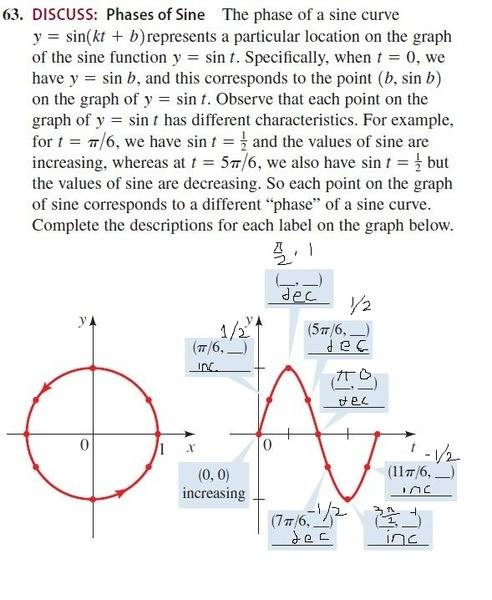# How to find out various sine values from its graph.

• BThe answer is written in thin black, inc = increasing, dec = decreasing. Am I wrong anywhere?

Thanks!

.Scott
Homework Helper
I would quibble over pi/2 and 3pi/2. In both of those cases the value of sin(x)neither increases nor decreases as x increases .

mfb
Mentor
I would quibble over pi/2 and 3pi/2. In both of those cases the value of sin(x)neither increases nor decreases as x increases .
Same here. Everything else is correct.

I would quibble over pi/2 and 3pi/2. In both of those cases the value of sin(x)neither increases nor decreases as x increases .
Yeah, I also spent more time on those than others. I can see that the derivative there would be a horizontal straight line. Fine, thank you!